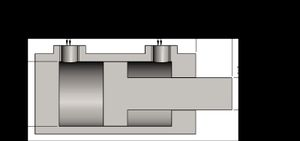# Hydraulic Unknown Cylinder Calculator

If the dimensions of a hydraulic cylinder are known, then numerous volumetric calculations can be carried out such as Full bore area and volumes.

If the hydraulic system pressure is also known then the Thrust Force and Pull Force can also be determined.

If the required cylinder speed is known then the Power required and Flow rates can also be calculated.

To use this calculator to find the FULL BORE VOLUME and CYLINDER BORE DIAMETER enter the

working pressure, total thrust force and number of cylinders and select units as required.

To use this calculator to find the PRESSURE enter the full bore volume,

total thrust force and number of cylinders and select units as required.

To use this calculator to find the SPEED enter the stroke and extend time and select units as required.### Affiliate Partners

Help support us by using our affiliate links below.